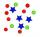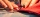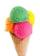# Calculator compound fraction

The calculator performs basic and advanced operations with fractions, expressions with fractions combined with integers, decimals, and mixed numbers. It also shows detailed step-by-step information about the fraction calculation procedure. Solve problems with two, three, or more fractions and numbers in one expression.

### 3/4 * 5/7 = 15/28 ≅ 0.5357143

Spelled result in words is fifteen twenty-eighths.

### How do you solve fractions step by step?

1. Multiple: 3/4 * 5/7 = 3 · 5/4 · 7 = 15/28
Multiply both numerators and denominators. Result fraction keep to lowest possible denominator GCD(15, 28) = 1. In the next intermediate step, the fraction result cannot be further simplified by canceling.
In words - three quarters multiplied by five sevenths = fifteen twenty-eighths.

#### Rules for expressions with fractions:

Fractions - use the slash “/” between the numerator and denominator, i.e., for five-hundredths, enter 5/100. If you are using mixed numbers, be sure to leave a single space between the whole and fraction part.
The slash separates the numerator (number above a fraction line) and denominator (number below).

Mixed numerals (mixed fractions or mixed numbers) write as non-zero integer separated by one space and fraction i.e., 1 2/3 (having the same sign). An example of a negative mixed fraction: -5 1/2.
Because slash is both signs for fraction line and division, we recommended use colon (:) as the operator of division fractions i.e., 1/2 : 3.

Decimals (decimal numbers) enter with a decimal point . and they are automatically converted to fractions - i.e. 1.45.

The colon : and slash / is the symbol of division. Can be used to divide mixed numbers 1 2/3 : 4 3/8 or can be used for write complex fractions i.e. 1/2 : 1/3.
An asterisk * or × is the symbol for multiplication.
Plus + is addition, minus sign - is subtraction and ()[] is mathematical parentheses.
The exponentiation/power symbol is ^ - for example: (7/8-4/5)^2 = (7/8-4/5)2

The calculator follows well-known rules for order of operations. The most common mnemonics for remembering this order of operations are:
PEMDAS - Parentheses, Exponents, Multiplication, Division, Addition, Subtraction.
BEDMAS - Brackets, Exponents, Division, Multiplication, Addition, Subtraction
BODMAS - Brackets, Of or Order, Division, Multiplication, Addition, Subtraction.
GEMDAS - Grouping Symbols - brackets (){}, Exponents, Multiplication, Division, Addition, Subtraction.
Be careful, always do multiplication and division before addition and subtraction. Some operators (+ and -) and (* and /) has the same priority and then must evaluate from left to right.

## Fractions in word problems:

• Ratio - proportionReduce the number 16 in proportion 3:2 5:4 11:8
• Dice and coinA number cube is rolled and a coin is tossed. The number cube and the coin are fair. What is the probability that the number rolled is greater than 2 and the coin toss is head?
• SewingBeth's mother can sew 235 pairs of short pants in 6 days while Lourdes can sew 187 pairs in 8 days. How many more pairs of short pants can Beth's mother sew?
• Youth trackYouth track from Hronská Dúbrava to Banská Štiavnica which announced cancellation attracted considerable media attention and public opposition, has cost 6.3 euro per capita and revenue 13 cents per capita. Calculate the size of subsidies to a trip group o
• Drinking waterA man drinks a keg of water in 40 days, and a woman drinks in 62 days. How many days do they consume a keg together?
• BonusThe gross wage was 1323 USD including 25% bonus. How many USD were bonuses?
• SomeoneSomeone ate 1/10 of a cake, leaving only 9/10. If you eat 2/3 of the cake that is left, how much of a whole cake will you have eaten?
• Fractions 3Calculate 1/9 of 27:
• There 12There are 42 students in the class and 2/3 of them are girls. How may girls are there in the class?
• Paper clipsMrs. Bright is organizing her office supplies. There are 5 open boxes of paper clips in her desk drawer. Each box has 1/2 of the paper clips remaining. How many boxes of paper clips are left?
• Multiplying fractionsWhat is 1/3 of 5?
• Ms. SheppardMs. Sheppard cuts ½ of a piece of construction paper. She uses ⅙ of the piece to make a flower. What fraction of the sheet of paper does she use to make the flower?
• IcecreamsKarthik bought 30 icecreams for his birthday .2/5 of them are chocolate icecreams. Find the number of chocolate icecreams he bought?